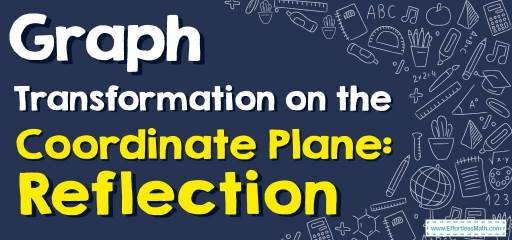# How to Graph Transformation on the Coordinate Plane: Reflection?

This article teaches you how to graph Reflections on the coordinate plane in a few simple steps.## Step by step guide to graph Transformation: Reflection

• Reflection is flipping an object across a line without changing its size or shape. So, a reflection is a mirror image of the shape. In this case, the image is a reflection of the pre-image and each point of the image is equidistant from each corresponding point in the pre-image.
• Reflecting the image over the $$x$$-axis to create a mirror image is called the reflection on the $$x$$-axis, and in this case, the $$x$$-axis is called the axis of reflection.
• Reflecting the image over the $$y$$-axis to create a mirror image is called the reflection on the $$y$$-axis, and in this case, the $$y$$-axis is called the axis of reflection.
• The reflection of the point $$(x, y)$$ across the $$x$$-axis is the point $$(x, -y)$$.
• The reflection of the point $$(x, y)$$ across the $$y$$-axis is the point $$(-x, y)$$.
• The reflection of the point $$(x, y)$$ across the line $$y=x$$ is the point $$(y, x)$$.
• The reflection of the point $$(x, y)$$ across the line $$y=-x$$ is the point $$(-y, -x)$$.
• When reflecting a point in the origin, both the $$x$$-coordinate and the $$y$$-coordinate is negated.$$(x, y)→(-x, -y)$$

### Transformation: Reflection – Example 1:

Graph the image of the figure using the transformation given. Reflection across the $$x$$-axis.

Solution:

Find the original coordinates:

$$A=(-3, 4)$$ $$B=(-3, 2)$$ $$C=(3, 2)$$

The reflection of the point $$(x, y)$$ across the $$x$$-axis is the point $$(x, -y)$$, So:

$$A^\prime=(-3, -4)$$ $$B^\prime=(-3, -2)$$ $$C^\prime=(3, -2)$$

The image of triangle $$ABC$$ is $$A^\prime B^\prime C^\prime$$ . (the mark $$^\prime$$ is called prime.)

### Transformation: Reflection – Example 2:

Graph the image of the figure using the transformation given. Reflection across the $$y$$-axis.

Solution:

Find the original coordinates:

$$A=(-3, 4)$$ $$B=(-4, 2)$$ $$C=(-2, -1)$$ $$D=(-1, 3)$$

The reflection of the point $$(x, y)$$ across the $$y$$-axis is the point $$(-x, y)$$, So:

$$A^\prime=(3, 4)$$ $$B^\prime=(4, 2)$$ $$C^\prime=(2, -1)$$ $$D^\prime=(1, 3)$$

The image of Polygon $$ABCِِD$$ is $$A^\prime B^\prime C^\prime D^\prime$$.

## Exercises for Transformation: Reflection

### Graph the image of the figure using the transformation given.

1. Reflection across line: $$y=x$$

2. Reflection across line: $$y=1$$

### What people say about "How to Graph Transformation on the Coordinate Plane: Reflection? - Effortless Math: We Help Students Learn to LOVE Mathematics"?

No one replied yet.

X
30% OFF

Limited time only!

Save Over 30%

SAVE $5 It was$16.99 now it is \$11.99